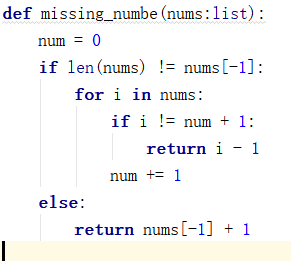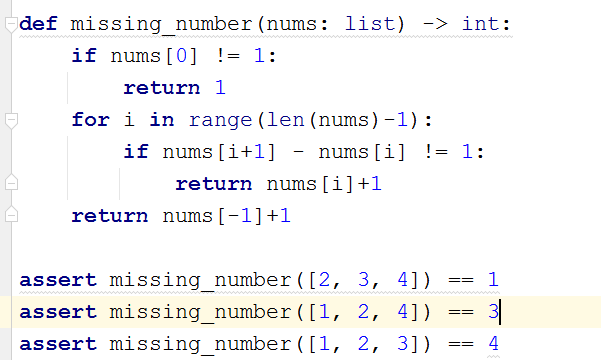# 【每日一题1020】失踪的数字给定一个连续的数字列表，元素从数字1开始。但是发现其中有且仅有一个数字失踪了。请编写一个函数，找出这个失踪的数字。

``````def missing_number(nums: list) -> int:
pass

assert missing_number([2, 3, 4]) == 1
assert missing_number([1, 2, 4]) == 3
assert missing_number([1, 2, 3]) == 4
``````
``````def missing_number(nums: list) -> int:
i = 1
for n in nums:
if n != i:
return i
i += 1
return i

assert missing_number([2, 3, 4]) == 1
assert missing_number([1, 2, 4]) == 3
assert missing_number([1, 2, 3]) == 4
``````
1 Like``````def missing_number(nums: list) -> int:
return [i for i in range(1,len(nums) +2) if i not in nums]

assert missing_number([2, 3, 4]) == 1
assert missing_number([1, 2, 4]) == 3
assert missing_number([1, 2, 3]) == 4
``````
``````from functools import reduce

def missing_number(nums: list) -> int:
nums.extend(list(range(1, len(nums)+2)))
data = reduce(lambda x,y:x ^ y, nums)
return data

assert missing_number([2, 3, 4]) == 1
assert missing_number([1, 2, 4]) == 3
assert missing_number([1, 2, 3]) == 4
``````

1. 首先，创造一个辅助列表，包含的是所有完整元素。
2. 然后，将这个列表的元素统统添加到缺少独苗到原列表。
3. 最后，利用归约函数reduce，将所有的元素互相进行异或^运算，因为它不是成对出现的，最终就会剩下缺失的数字了。

@Mrhjs 同学的题解很棒！为了方便社区交流，后续题解建议使用下面这种markdown格式哦：

```python

```

``````def missing_number(nums: list) -> int:
return sum(range(1,len(nums)+2))-sum(nums)

assert missing_number([2, 3, 4]) == 1
assert missing_number([1, 2, 4]) == 3
assert missing_number([1, 2, 3]) == 4
``````

3 Likes
``````def missing_number(nums: list) -> int:
if nums != 1:
return 1
else:
for i in nums:
if i + 1 not in nums:
return i + 1

assert missing_number([2, 3, 4]) == 1
assert missing_number([1, 2, 4]) == 3
assert missing_number([1, 2, 3]) == 4
``````
``````    public int missingNumber(ArrayList arrayList){
for (int i = 1; i <= arrayList.size()+1; i++) {
if (arrayList.indexOf(i) == -1){
return i;
}
}
return -1;
}
``````
``````def missing_number(nums: list) -> int:
l = [i+1 for i in range(len(nums) + 1)]
l2 =[i for i in l if i not in nums]
return l2

assert missing_number([2, 3, 4]) == 1
assert missing_number([1, 2, 4]) == 3
assert missing_number([1, 2, 3]) == 4
``````
``````def missing_number(nums: list) -> int:
index = 0
while index<len(nums)-1:
if nums[index]+1!=nums[index+1]:
return nums[index]+1
index+=1
if nums-1>0:
return nums-1
else:
return nums[-1]+1``````
``````def missing_number(nums: list) -> int:
for i in range(1,len(nums)+1):
if i not in nums: return i
return len(nums)+1
``````
``````def solution(nums: list):
for i in range(0, len(nums)):
if i == 0:
if nums > 1:
return nums - 1
continue
elif nums[i] == nums[i-1] + 1:
continue
else:
return nums[i] - 1
return nums[-1] + 1

assert solution([2, 3, 4]) == 1
assert solution([1, 2, 4]) == 3
assert solution([1, 2, 3]) == 4
````````````def missing_number(nums: list) -> int:
if nums != 1:
return 1
else:
for i in range(len(nums)-1):
if  nums[i] + 1 != nums[i+1]:
return nums[i] + 1
else:
return nums[-1] + 1
``````